## 1. Two wires – A and B – with circular cross-sections have identical lengths and are made of the same material. Yet, wire A has four times t

Question

1. Two wires – A and B – with circular cross-sections have identical lengths and are made of the same material. Yet, wire A has four times the resistance of wire B. How many times greater is the diameter of wire B than wire A?

in progress 0
5 months 2021-08-05T00:53:01+00:00 1 Answers 105 views 0

## Answers ( )

Diameter of wire B is 2 times the diameter of wire A

Explanation:

We have given two wire A and B

They are made up of same material and length of both the wire is sane

So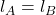Let the resistivity of both the wire is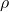It is given that wire A has 4 times the resistance as wire B

So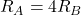So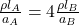( As)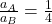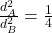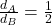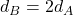So diameter of wire B is 2 times the diameter of wire A